•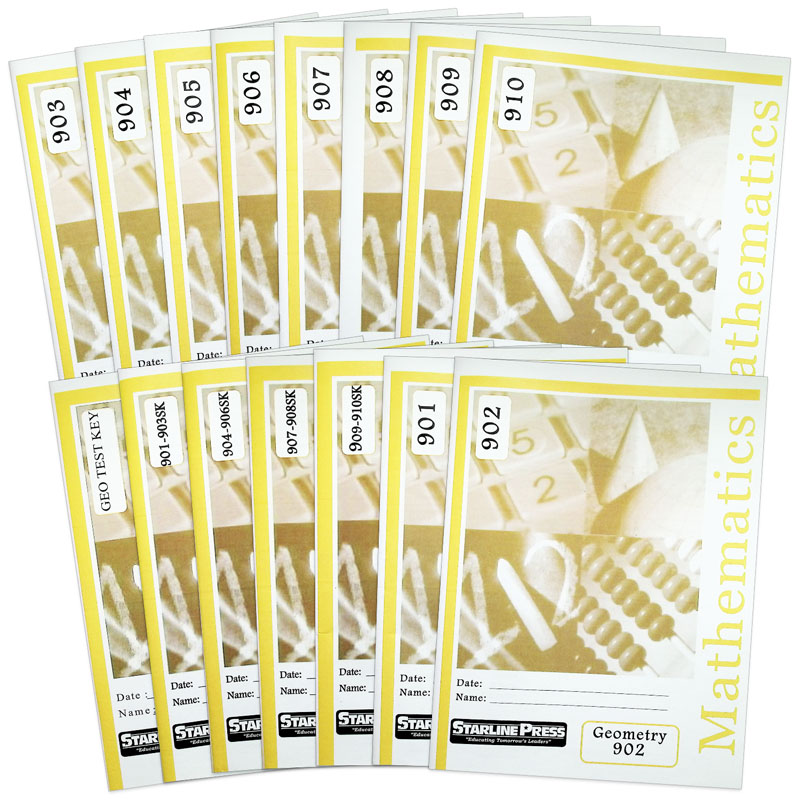•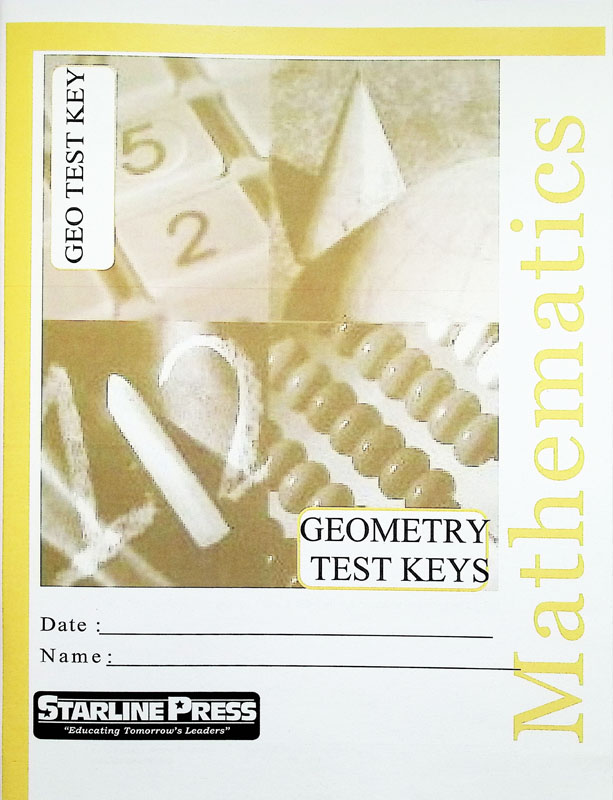•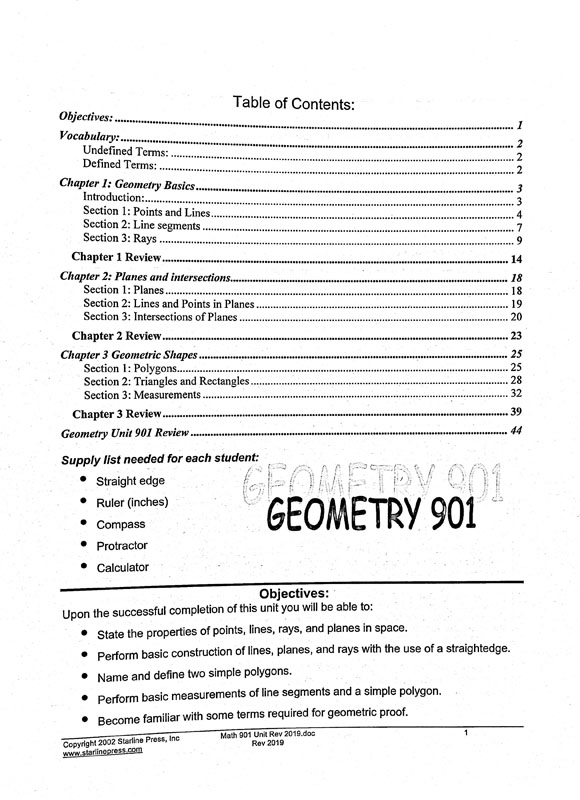•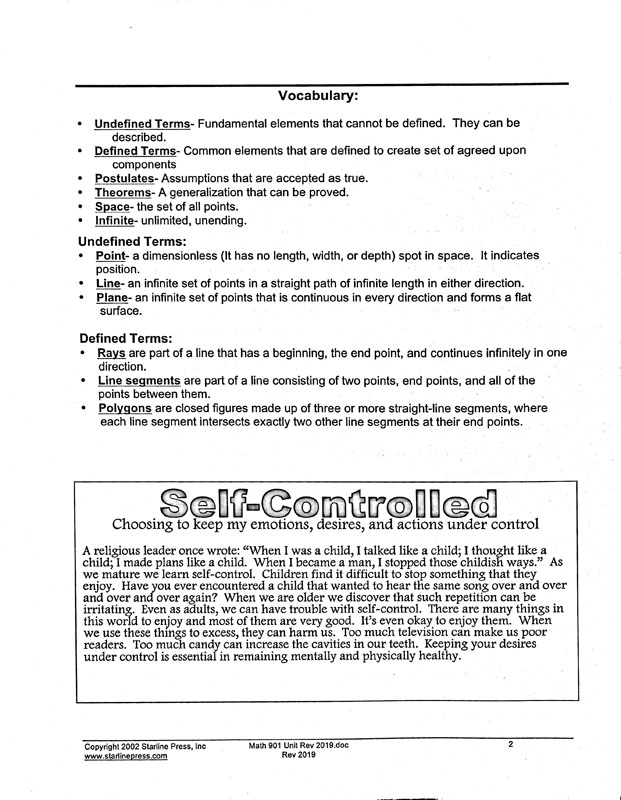•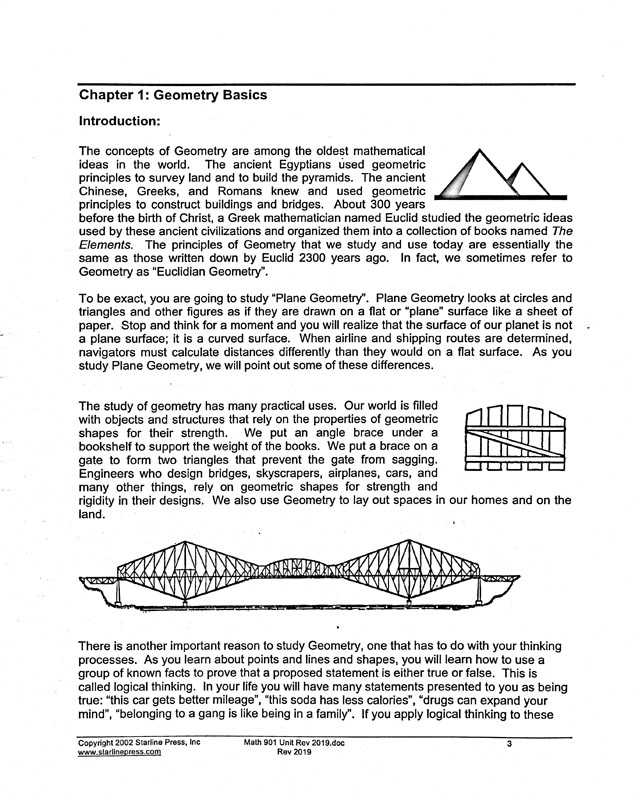# Geometry Set

# 051457

Our Price: \$175.95
Retail: \$186.72
Save: 5.77% (\$10.77)
In Stock.
Qty:
Qty:

 Item #: 051457 9

#### Product Description:

GEOMETRY: Covers Geometry basics: points and lines, line segments, rays, planes, and lines and points in planes. Students study definitions of angles, degrees, and measuring the size of angles. They are taught to classify angles by size and relation and how to bisect an angle. Students study parallelograms, properties of a trapezoid, ration and proportions, and the Pythagorean Theorem. Ninth grade students know, derive, and solve problems involving perimeter, circumference, area, volume, lateral area and surface area of common geometric features. Students know the definitions of basic trigonometric functions defined by the angles of a right triangle. They also know and are able to use elementary relationships between functions.

#### Publisher Description:

Ninth grade students learn points and lines, line segments, rays, planes, and lines and points in planes. They study definitions of angles and degrees and measuring the size of angles. They learn to classify angles by size and relation and how to bisect an angle. Students study parallelograms, properties of a trapezoid, ratio and proportions, and the Pythagorean Theorem. Ninth grade students know derive and solve problems involving the perimeter, circumference, area, volume, lateral area, and surface area of common geometric figures. Students know the definitions of basic trigonometric functions defined by the angles of a right triangle. They also know and are able to use elementary relationships between functions.

Category Description for Starline Press Math:

Starline Press Math is a character-based, individualized and independent learning curriculum. Aligned to pre-Common Core California state standards (please see publisher website for CA state standards correlation), each grade level provides self- directed instruction with minimal teacher supervision. Grades 3-8 complete twelve units (booklets) per year, with a suggested 3 week completion time frame. Grades 9-10 complete 6 booklets per semester /12 per year. Each grade level includes vocabulary, fill in the blank questions, practice problems, chapter reviews, and unit tests. Consumable worktexts are colorfully illustrated soft covers, although newer printings are more like booklets and do not have the perfect binding and glossy covers. Available only in complete sets which include answer and test keys. ~ Deanne

Category Description for COMPREHENSIVE PROGRAMS - ALL GRADES:

Primary Subject
Mathematics
9
Format
Softcover Book Set
Brand Name
Starline Press
Weight
4.7 (lbs.)
Dimensions
11.0" x 8.5" x 2.5"